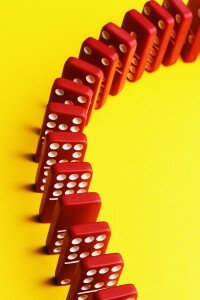# Decimal ActivitiesEven kids use decimals almost everyday without even realizing it. They speak of dollars and cents in everyday conversation, and are using one of the applications of decimals. Yet for some reason, this remains one area of math that elementary students find intimidating. It doesn’t have to be that way. Here are several creative methods of teaching about tenths and hundredths that your learners will find simple and actually enjoy.

1) Kids seem to have trouble saying decimals correctly. The Human Decimal Machine is a way of helping them. On pieces of construction paper, write the digits 0 through 9, and another one with a decimal point, and give one to each learner to represent the card. Tell them the way each one says the decimal point is using the word “and.” Tell them that whoever is to the right of the decimal must be called either “tenths” or “hundredths” or “thousandths,” etc. Have different kids with their cards rearrange themselves and then the other kids read the decimal correctly. For instance, if the persons holding the 9, the decimal and the 5, stand before the class, the kids should read it respectively, as ‘Nine’ ‘and’ ‘five’ ‘tenths’.

2) Using money is one of the most intuitive ways to teach about decimals. Divide your kids into three groups. Give one group play money and coins, give another group a play / cut-out “pie”, and give a third group some dominos. The idea here is to go from the known to the unknown. Most kids know what a dollar looks like, what a half dollar is, and what a “quarter” dollar is. (Explain that a quarter is another way of saying “one fourth” or “one out of four”.) Begin by showing visually that two half dollars make one whole dollar; four quarter dollars — or four “fourth dollars” make one whole dollar, etc. Now translate to non-monetary items: If there are two “half dollars” in a whole, then how many half-pies are in a whole? Use dominoes to illustrate similar points. After students understand the easy ones, move one to dimes, explaining that they are “hundredths.”

3) Decimal Bingo. This only works for kids who have just learned fractions. Cover your Bingo boards with decimals. When you call out the spaces, call them out as fractions. They must locate the decimal equivalent to cover that spot on their board.

4) For an idea for using money to teach decimals, make up a realistic restaurant menu. Tell the students to order what they want, but they have to add up how much money they’ll need. This fun exercise involves the kids in adding decimals.

You’ll find that these four ideas will have your elementary kids looking forward to their decimal lessons.

Here are some of our fun learning games On Decimals:
http://www.math-lessons.ca/activities/OneEquals.html (decimals, fractions, percentiles and money)
http://www.math-lessons.ca/Decimals/decimal.html
http://www.math-lessons.ca/Decimals/decimalBoard4.html